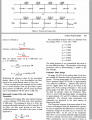# Second order passive low pass filter design

#### Ian0

Joined Aug 7, 2020
6,983
Handbook of Filter Synthesis
Anatol I. Zverev
ISBN 10: 0471749427ISBN 13: 9780471749424

It consists of nothing more than the tables, and graphs of impulse responses. It tells you nothing about filter design.
Its the sort of book that you look at in the library, take a copy of the table that you need and put back!
There are much better books about filter design.
Analog Filter Design by van Valkenburg often gets recommended on this forum (usually by @Papabravo )
but no matter how much you read, you are not going to design the filter you want using a smaller value of inductance. Unless you take note of the experts’ comments you will be stuck at the same stage of your design in a year’s time.

•pinkyponky

#### pinkyponky

Joined Nov 28, 2019
318
$$L_1 = \frac{k R_l}{2\pi f}$$
where k is the coefficient you have read from the table, f is the cutoff frequency, and Rl is the load impedance.
Same for the capacitor
$$C_1 = \frac{k}{2\pi fR_l}$$
The above L and C formulas are assumed from the below image which is from the Handbook of Filter Synthesis.Here, I have some questions on above formulas.

How you add 'K' as a coefficient in the above formals. And also how you consider Rr as a Load resistance?.
In the book stated that the ratio of the source to load impedance (Rs/Rl), but you posted reverse as (Rl/Rs), why?

Just want to understand.

Last edited:

#### Ian0

Joined Aug 7, 2020
6,983
The coefficients are the value in Farad or Henry which would be required for a filter with 1Ω load and a cutoff frequency of 1 rad/s

So, divide by the frequency in rad/s (which is 2πf)
Then multiply inductors by the load resistance or divide capacitors by the load resistance.
That produces the formula I gave you.
The load resistor goes between the output terminal and 0V
The source resistance goes in series with the input terminal.
Generally the source resistance is zero.

#### pinkyponky

Joined Nov 28, 2019
318
The coefficients are the value in Farad or Henry which would be required for a filter with 1Ω load and a cutoff frequency of 1 rad/s

So, divide by the frequency in rad/s (which is 2πf)
Then multiply inductors by the load resistance or divide capacitors by the load resistance.
That produces the formula I gave you.
The load resistor goes between the output terminal and 0V
The source resistance goes in series with the input terminal.
Generally the source resistance is zero.
Why the coefficient value is only multiplying in both equation?. why can't it divide?.
Why can't we consider Rr as a Source resistance why Load resistance?.
Why K is missing in the formula in the page which I have attached in the post #222?.
In the book stated that the ratio of the source to load impedance (Rs/Rl), but you posted reverse as (Rl/Rs), why?

#### Ian0

Joined Aug 7, 2020
6,983
Why the coefficient value is only multiplying in both equation?. why can't it divide?.
Why can't we consider Rr as a Source resistance why Load resistance?.
Why K is missing in the formula in the page which I have attached in the post #222?.
In the book stated that the ratio of the source to load impedance (Rs/Rl), but you posted reverse as (Rl/Rs), why?
It’s a long time since I used the tables, but I think high-pass designs divide.
Not sure I know what you mean about source and load resistance.
In the text he says that you work out a “base” capacitance and inductance value, and then multiply that by the coefficients.
He refers to working out the base value as “denormalising” the filter. A “normalised” filter is one with a 1Ω load resistance and a cutoff frequency of 1 rad/s.
The Rs/Rl is used the other way round if it is a current-fed filter, that is the parallel component comes first - a π-section filter rather than a T-section filter if it were third order.

#### BobTPH

Joined Jun 5, 2013
6,293
Hi Bob,

I gave the full details such as input resistance, load resistance, application of the filter circuit design, and also I have derive the transfer function. I struck here that reduce the inductance value.
Let’s say I gave all the same specifications for my car except the size of the fuel tank. Then the car can be made easily. You did exactly the same thing with your filter. There is nothing wrong with your specifications until you get to the size of the inductor.